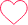# Python Program for Printing Inverted Hollow Pyramid Star Pattern

## Print Inverted Hollow Pyramid Star Pattern

Let’s look at the program for hollow inverted right triangle star pattern in python.

```Enter the Number: 7
*************
*         *
*       *
*     *
*   *
* *
*
```## Hollow inverted right triangle star pattern in python

### Working:

• Step 1. Start
• Step 2. Take number of rows as input from the user and stored it into num.
• Step 3. Run a loop ‘i’ number of times to iterate through all the rows which is Starting from i=0 to num.
• Step 4. Run a nested loop inside the main loop for printing spaces which is starting from j=0 to i.
• Step 5. Run a nested loop inside the main loop for printing stars which is starting from j=num*2 to (num*2 – (2*i – 1))+1.
• Step 6. Inside the above loop print stars only if i == 1 or j == 1 or j ==(num*2 -(2*i-1)) in all other cases print a blank space.
• Step 7. Move to the next line by printing a new line using print() function.
• Step 8. Stop

### Python Program:

Run
```num = int(input("Enter the Number: "))

for i in range(1, num+1):
for j in range(0, i):
print(" ", end="")

for j in range(1, (num*2 - (2*i - 1))+1):
if i == 1 or j == 1 or j ==(num*2 -(2*i-1)):
print("*", end="")
else:
print(" ", end="")
print()
```

### Output

```Enter the Number: 7
*************
*         *
*       *
*     *
*   *
* *
*

Process finished with exit code 0
```

### 5 comments on “Python Program for Printing Inverted Hollow Pyramid Star Pattern”

•Siva sankar reddy Asam

TRY THIS BRO, IT’S WAY EASIER;
n = int(input(‘enter a number: ‘))

for i in range(n):
if i == 0:
print(‘*’*(n*2-1))
elif i == n-1:
print(‘ ‘*(n-1) + ‘*’)
else:
print(‘ ‘*i + ‘*’ + ‘ ‘*(2*(n-1-i)-1) + ‘*’)0
•PrepInsta Support0
•Arpita

n=int(input(‘Enter number:’))
for i in range(n):
for j in range(i):
print(‘ ‘,end=”)
for j in range(i,(2*n)-(i+1)):
if j==i or i==0 or j==(2*n)-(i+2):
print(‘*’,end=”)
else:
print(‘ ‘,end=”)
print()0
•# Inverted Hollow Pyramid Star Pattern Method – 2

n=int(input(“Enter the number: “))
for i in range(1,n+1):
for j in range(1,2*n):

# Condition of the if function
if(i==1 and j%2!=0) or i==j or i+j==n*2:
print(“*”,end=””)
else:
print(” “,end=””)
print()0
•Khushboo

n = int((input(“Enter the number:)))
for i in range(n,-1,-1):
if ((i==0) or (i==n)):
print(” “*(n-i)+ (“*”)*(2*i+1))
else:
print(” “*(n-i) + (“*”) + (” “)*(2*i -1) + (“*”))0